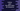# How to print the ASCII values all characters in Python## How to print the ASCII values all characters in Python:

We can use Python to print the ASCII values of all characters. We can run a loop to iterate through the alphabet characters and print the ASCII values.

We will learn how to print the lowercase and uppercase characters and ASCII values of these characters using python.

### string module:

string is an inbuilt module of Python. It provides different constants. We can use the ascii_lowercase and ascii_uppercase for this example. ascii_lowercase is a string containing all lower-case characters of engligh alphabet and ascii_uppercase is a string containing upper-case characters.

We can use a for loop to iterate through the character of these strings.

### ord() function:

ord() function takes one character as the parameter and returns the unicode for that character. This function can be used to print the ASCII value of a character in Python.

### Python program to print the ASCII values of all lowercase characters:

The below python program prints the ASCII values of all lowercase characters:

``````import string

for c in string.ascii_lowercase:
print(f'ASCII for {c} is {ord(c)}')``````

If you run this program, it will print:

``````ASCII for a is 97
ASCII for b is 98
ASCII for c is 99
ASCII for d is 100
ASCII for e is 101
ASCII for f is 102
ASCII for g is 103
ASCII for h is 104
ASCII for i is 105
ASCII for j is 106
ASCII for k is 107
ASCII for l is 108
ASCII for m is 109
ASCII for n is 110
ASCII for o is 111
ASCII for p is 112
ASCII for q is 113
ASCII for r is 114
ASCII for s is 115
ASCII for t is 116
ASCII for u is 117
ASCII for v is 118
ASCII for w is 119
ASCII for x is 120
ASCII for y is 121
ASCII for z is 122``````

### Python program to print the ASCII values of all uppercase characters:

In a similar way, we can also print the ASCII values of all uppercase characters.

``````import string

for c in string.ascii_uppercase:
print(f'ASCII for {c} is {ord(c)}')``````

It will print:

``````ASCII for A is 65
ASCII for B is 66
ASCII for C is 67
ASCII for D is 68
ASCII for E is 69
ASCII for F is 70
ASCII for G is 71
ASCII for H is 72
ASCII for I is 73
ASCII for J is 74
ASCII for K is 75
ASCII for L is 76
ASCII for M is 77
ASCII for N is 78
ASCII for O is 79
ASCII for P is 80
ASCII for Q is 81
ASCII for R is 82
ASCII for S is 83
ASCII for T is 84
ASCII for U is 85
ASCII for V is 86
ASCII for W is 87
ASCII for X is 88
ASCII for Y is 89
ASCII for Z is 90``````